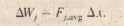# Work Done by a General Variable Force

Work Done by a General Variable Force

One-Dimensional Analysis

Let us return to the situation of Fig. 7-2 but now consider the force to be directed along the x axis and the force magnitude to vary with position x. Thus, as the bead (particle) moves, the magnitude of the force doing work on ft changes. Only the magnitude of this variable force changes, not its direction, and the magnitude at any position does not change with time.

With considered constant. the increment (small amount) of work done by the force in the  interval is now approximately given byWe can make the approximation better by reducing the strip width and using more strips. as in . In the limit, we let the strip width approach zero: the number of strips then becomes infinitely large and we have, as an exact result.This limit is exactly what we mean by the integral of the function F(x) between the limits.If we know the function F(x), we can substitute it into , introduce the proper limits of integration. carry out the integration. and thus find the work. (Appendix   contains a list of common integrals.) Geometrically.a) A one-dimensional force fploucd against the displacement .r of aparticle on which it acts. The particlemoves from x, to Xj. (h) Same as (a)but with the area under the curve dividedinto narrow strips. (e) Same as(h) but with the area divided into narrowerstrips. (d) The limiting case. Thework done by the force is given by and is represented by the shadedarea between the curve and the x axisand between.I; and Xi.

Three-Dimensional Analyses

Consider now a particle that is acted on by a three-dimensional forceThe increment of work  done on the particle by F during the displacement d?The work W done by F while the particle moves from an initial position with coordinates (x,y. z) to a final position  with coordinates.If F has only an x component, then the y and z terms in are zero and the equation reduces.

Work-Kinetic Energy Theorem with a Variable Force

Equation 7-32 gives the work done by a variable force on a particle in a one dimensional
situation. Let us now make certain that the work calculated with  is indeed equal to the change in kinetic energy of the particle, as the work kinetic energy theorem states.which we use Newton’s second law to replace F(x) with ma. We can write the quantity.Note that when we change the variable from x to v we are required to express the limits on the integral in terms of the new variable. Note also that because the mass m is a constant, we are able to move it outside the integral.